# How to find Percentage Change in pandas

So you are interested to find the percentage change in your data. Well it is a way to express the change in a variable over the period of time and it is heavily used when you are analyzing or comparing the data. In this post we will see how to calculate the percentage change using pandas pct_change() api and how it can be used with different data sets using its various arguments.

As per the documentation, the definition of pandas pct_change method and its parameters are as shown:

pct_change_(self, periods=1, fill_method=’pad’, limit=None, freq=None,) _

periods : int, default 1 Periods to shift for forming percent change.

fill_method : str, default ‘pad’ How to handle NAs before computing percent changes.

limit : int, default None The number of consecutive NAs to fill before stopping.

freq : DateOffset, timedelta, or offset alias string, optional Increment to use from time series API (e.g. ‘M’ or BDay())

Before we dive deeper into using the pct_change, Lets understand how the Percentage change is calculated across the rows and columns of a dataframe

### Create a Dataframe

``````import pandas as pd
import random
import numpy as np

df = pd.DataFrame({"A":[1,4,5,4,6,10,14,None,20,22],
"B":np.random.uniform(low=10.5, high=45.3, size=(10,)),
"C":np.random.uniform(low=70.5, high=85, size=(10,))})
df
``````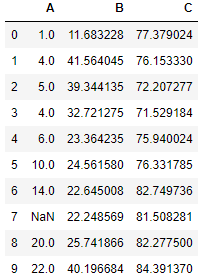## Percentage Change between rows

Here we will find out the percentage change between the rows. We are interested to find out the pct change in value for all indexes across the columns A,B and C. For example: percentage change between Column A and B at index 0 is given by the following formula: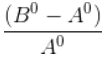Where B0 is value of column B at index 0 and A0 is value at column A.

``````df.pct_change(axis=1)
``````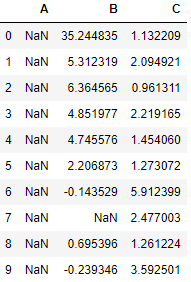## Percentage Change between two columns

The first row will be NaN since that is the first value for column A, B and C. The percentage change between columns is calculated using the formula: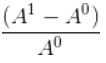Where A1 is value of column A at index 0 and A1 is value at index 1

``````df.pct_change(axis=0,fill_method='bfill')
``````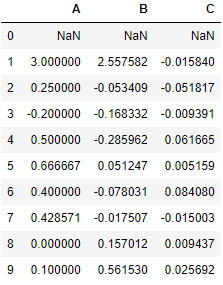## fill_method in pct_change

This is used to fill the NaN values in the data, there are two options i.e. pad and bfill that you can select to fill the NaN values in your data , By default it is `pad`, which means the NaN values in the data will be filled by the value from preceding row or column whereas `bfill` which stands for backfill means the NaN values will be filled by the value from succeeding row or column values.

There is another argument `limit` which is used to decide how many NaN values you want to fill using these methods

## Percentage Change for Time series data

In our time-series data we have the date index with a daily frequency.

``````import pandas as pd
import random
import numpy as np

# Creating the time-series index
n=92
index = pd.date_range('01/01/2020', periods = n,freq='D')

# Creating the dataframe
df = pd.DataFrame({"A":np.random.uniform(low=0.5, high=13.3, size=(n,)),
"B":np.random.uniform(low=10.5, high=45.3, size=(n,)),
"C":np.random.uniform(low=70.5, high=85, size=(n,)),
"D":np.random.uniform(low=50.5, high=65.7, size=(n,))}, index = index)

``````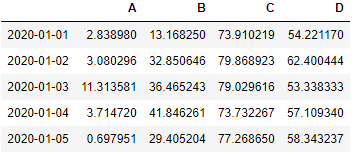## freq in pct_change()

So using the `freq` argument you can find the percentage change for any timedelta values, Suppose using this dataframe you want to find out the percentage change after every 5 days then set the freq as 5D. The first five rows is NaN since there are no 5 days back data is present for these values to find the pct change. Only we can start with the 6th row which can be compared with the 1st row to find the pct change for 5 days and similarly we can get pct_change for following rows

``````df.pct_change(freq='5D')
``````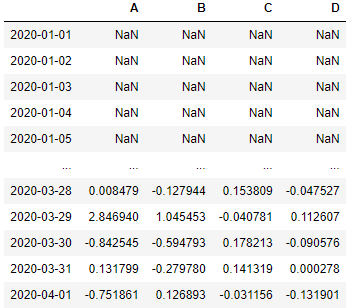## Monthly pct_change() in time series data

With the same time-series lets find out how to find the monthly pct change in these values. First we need to get the Data for the last day of each month. So we will resample the data for frequency conversion and set the rule as ‘BM’ i.e. Business Month.

``````monthly = df.resample('BM', how=lambda x: x[-1])
``````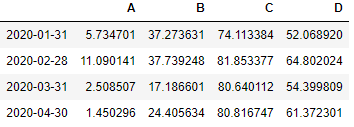Now apply the pct_change() on this data to find out the monthly percentage change

``````monthly.pct_change()
``````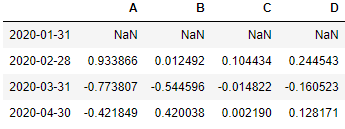if you want the monthly percentage change for the months which has only the last day date available

``````df.asfreq('BM').pct_change()
``````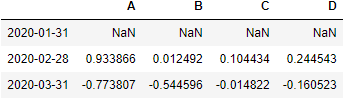## pct_change in groupby

You can also find the percentage change within each group by applying pct_change() on the groupby object. The first value under pct_change() for each group is NaN since we are interested to find the percentage change within each group only.

``````df = pd.DataFrame({'Name': ['Ali', 'Ali', 'Ali', 'Cala', 'Cala', 'Cala', 'Elena', 'Elena', 'Elena'],
'Time': [1, 2, 3, 1, 2, 3, 1, 2, 3],
'Amount': [24, 52, 34, 95, 98, 54, 32, 20, 16]})
``````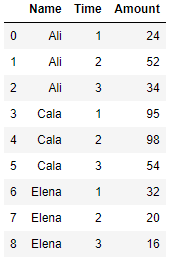``````df['pct_change'] = df.groupby(['Name'])['Amount'].pct_change()
df
``````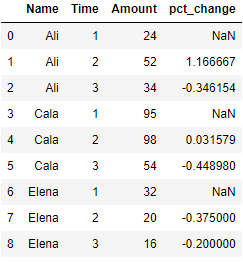Tags:

Categories:

Updated: